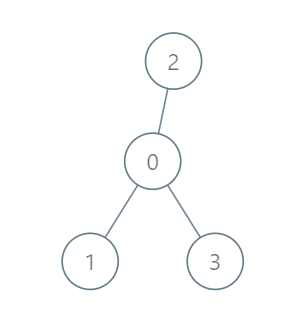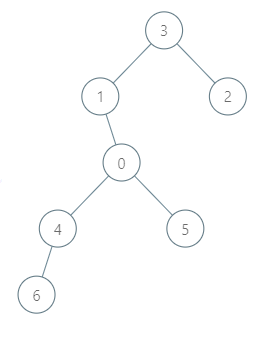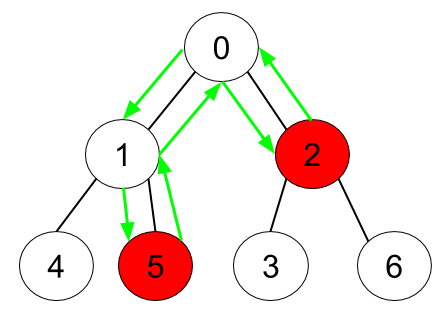## C# || How To Find The Minimum Fuel Cost To Report To The Capital Using C#The following is a module with functions which demonstrates how to find the minimum fuel cost to report to the capital using C#.

1. Minimum Fuel Cost – Problem Statement

There is a tree (i.e., a connected, undirected graph with no cycles) structure country network consisting of n cities numbered from 0 to n – 1 and exactly n – 1 roads. The capital city is city 0. You are given a 2D integer array roads where roads[i] = [ai, bi] denotes that there exists a bidirectional road connecting cities ai and bi.

There is a meeting for the representatives of each city. The meeting is in the capital city.

There is a car in each city. You are given an integer seats that indicates the number of seats in each car.

A representative can use the car in their city to travel or change the car and ride with another representative. The cost of traveling between two cities is one liter of fuel.

Return the minimum number of liters of fuel to reach the capital city.

Example 1:``` Input: roads = [[0,1],[0,2],[0,3]], seats = 5 Output: 3 Explanation: - Representative1 goes directly to the capital with 1 liter of fuel. - Representative2 goes directly to the capital with 1 liter of fuel. - Representative3 goes directly to the capital with 1 liter of fuel. It costs 3 liters of fuel at minimum. It can be proven that 3 is the minimum number of liters of fuel needed. ```

Example 2:``` Input: roads = [[3,1],[3,2],[1,0],[0,4],[0,5],[4,6]], seats = 2 Output: 7 Explanation: - Representative2 goes directly to city 3 with 1 liter of fuel. - Representative2 and representative3 go together to city 1 with 1 liter of fuel. - Representative2 and representative3 go together to the capital with 1 liter of fuel. - Representative1 goes directly to the capital with 1 liter of fuel. - Representative5 goes directly to the capital with 1 liter of fuel. - Representative6 goes directly to city 4 with 1 liter of fuel. - Representative4 and representative6 go together to the capital with 1 liter of fuel. It costs 7 liters of fuel at minimum. It can be proven that 7 is the minimum number of liters of fuel needed. ```

Example 3:``` Input: roads = [], seats = 1 Output: 0 Explanation: No representatives need to travel to the capital city. ```

2. Minimum Fuel Cost – Solution

The following is a solution which demonstrates how to find the minimum fuel cost to report to the capital.

``` 2. Minimum Fuel Cost - Solution C# // ============================================================================ // Author: Kenneth Perkins // Date: May 24, 2023 // Taken From: http://programmingnotes.org/ // File: Solution.cs // Description: Demonstrates how to find minimum fuel cost to the capital // ============================================================================ public class Solution { public long MinimumFuelCost(int[][] roads, int seats) { int n = roads.Length + 1; var adj = new Dictionary<int, List<int>>(); int[] degree = new int[n]; foreach (int[] road in roads) { if (!adj.ContainsKey(road)) { adj[road] = new List<int>(); } adj[road].Add(road); if (!adj.ContainsKey(road)) { adj[road] = new List<int>(); } adj[road].Add(road); ++degree[road]; ++degree[road]; } return BFS(n, adj, degree, seats); } public long BFS(int n, Dictionary<int, List<int>> adj, int[] degree, int seats) { var q = new Queue<int>(); for (int i = 1; i < n; i++) { if (degree[i] == 1) { q.Enqueue(i); } } int[] representatives = new int[n]; Array.Fill(representatives, 1); long fuel = 0; while (q.Count > 0) { int node = q.Dequeue(); fuel += (long)Math.Ceiling((double) representatives[node] / seats); foreach (int neighbor in adj[node]) { --degree[neighbor]; representatives[neighbor] += representatives[node]; if (degree[neighbor] == 1 && neighbor != 0) { q.Enqueue(neighbor); } } } return fuel; } }// http://programmingnotes.org/ 12345678910111213141516171819202122232425262728293031323334353637383940414243444546474849505152535455565758 // ============================================================================//    Author: Kenneth Perkins//    Date:   May 24, 2023//    Taken From: http://programmingnotes.org///    File:  Solution.cs//    Description: Demonstrates how to find minimum fuel cost to the capital// ============================================================================public class Solution {    public long MinimumFuelCost(int[][] roads, int seats) {        int n = roads.Length + 1;        var adj = new Dictionary<int, List<int>>();        int[] degree = new int[n];         foreach (int[] road in roads) {            if (!adj.ContainsKey(road)) {                adj[road] = new List<int>();            }            adj[road].Add(road);             if (!adj.ContainsKey(road)) {                adj[road] = new List<int>();            }            adj[road].Add(road);             ++degree[road];            ++degree[road];        }         return BFS(n, adj, degree, seats);    }     public long BFS(int n, Dictionary<int, List<int>> adj, int[] degree, int seats) {        var q = new Queue<int>();        for (int i = 1; i < n; i++) {            if (degree[i] == 1) {                q.Enqueue(i);            }        }         int[] representatives = new int[n];        Array.Fill(representatives, 1);        long fuel = 0;         while (q.Count > 0) {            int node = q.Dequeue();            fuel += (long)Math.Ceiling((double) representatives[node] / seats);             foreach (int neighbor in adj[node]) {                --degree[neighbor];                representatives[neighbor] += representatives[node];                if (degree[neighbor] == 1 && neighbor != 0) {                    q.Enqueue(neighbor);                }            }        }        return fuel;    }}// http://programmingnotes.org/ ```

QUICK NOTES:
The highlighted lines are sections of interest to look out for.

The code is heavily commented, so no further insight is necessary. If you have any questions, feel free to leave a comment below.

Once compiled, you should get this as your output for the example cases:

``` 3 7 0 ```

## C# || How To Find The Minimum Time To Collect All Apples In A Tree Using C#The following is a module with functions which demonstrates how to find the minimum time to collect all apples in a tree using C#.

1. Min Time – Problem Statement

Given an undirected tree consisting of n vertices numbered from 0 to n-1, which has some apples in their vertices. You spend 1 second to walk over one edge of the tree. Return the minimum time in seconds you have to spend to collect all apples in the tree, starting at vertex 0 and coming back to this vertex.

The edges of the undirected tree are given in the array edges, where edges[i] = [ai, bi] means that exists an edge connecting the vertices ai and bi. Additionally, there is a boolean array hasApple, where hasApple[i] = true means that vertex i has an apple; otherwise, it does not have any apple.

Example 1:``` Input: n = 7, edges = [[0,1],[0,2],[1,4],[1,5],[2,3],[2,6]], hasApple = [false,false,true,false,true,true,false] Output: 8 Explanation: The figure above represents the given tree where red vertices have an apple. One optimal path to collect all apples is shown by the green arrows. ```

Example 2:``` Input: n = 7, edges = [[0,1],[0,2],[1,4],[1,5],[2,3],[2,6]], hasApple = [false,false,true,false,false,true,false] Output: 6 Explanation: The figure above represents the given tree where red vertices have an apple. One optimal path to collect all apples is shown by the green arrows. ```

Example 3:

``` Input: n = 7, edges = [[0,1],[0,2],[1,4],[1,5],[2,3],[2,6]], hasApple = [false,false,false,false,false,false,false] Output: 0 ```

2. Min Time – Solution

The following is a solution which demonstrates how to find the cheapest flights within K stops.

``` 2. Min Time - Solution C# // ============================================================================ // Author: Kenneth Perkins // Date: May 23, 2023 // Taken From: http://programmingnotes.org/ // File: Solution.cs // Description: Demonstrates how to find minimum time to collect apples // ============================================================================ public class Solution { public int MinTime(int n, int[][] edges, IList<bool> hasApple) { var adj = new Dictionary<int, List<int>>(); foreach (int[] edge in edges) { int a = edge; int b = edge; if (!adj.ContainsKey(a)) { adj[a] = new List<int>(); } if (!adj.ContainsKey(b)) { adj[b] = new List<int>(); } adj[a].Add(b); adj[b].Add(a); } return DFS(0, -1, adj, hasApple); } public int DFS(int node, int parent, Dictionary<int, List<int>> adj, IList<bool> hasApple) { if (!adj.ContainsKey(node)) { return 0; } int totalTime = 0; foreach (int child in adj[node]) { if (child == parent) { continue; } int childTime = DFS(child, node, adj, hasApple); // childTime > 0 indicates subtree of child has apples. Since the root node of the // subtree does not contribute to the time, even if it has an apple, we have to check it // independently. if (childTime > 0 || hasApple[child]) { totalTime += childTime + 2; } } return totalTime; } }// http://programmingnotes.org/ 12345678910111213141516171819202122232425262728293031323334353637383940414243444546 // ============================================================================//    Author: Kenneth Perkins//    Date:   May 23, 2023//    Taken From: http://programmingnotes.org///    File:  Solution.cs//    Description: Demonstrates how to find minimum time to collect apples// ============================================================================public class Solution {    public int MinTime(int n, int[][] edges, IList<bool> hasApple) {        var adj = new Dictionary<int, List<int>>();        foreach (int[] edge in edges) {            int a = edge;            int b = edge;            if (!adj.ContainsKey(a)) {                adj[a] = new List<int>();            }            if (!adj.ContainsKey(b)) {                adj[b] = new List<int>();            }            adj[a].Add(b);            adj[b].Add(a);        }        return DFS(0, -1, adj, hasApple);    }     public int DFS(int node, int parent, Dictionary<int, List<int>> adj, IList<bool> hasApple) {        if (!adj.ContainsKey(node)) {            return 0;        }         int totalTime = 0;        foreach (int child in adj[node]) {            if (child == parent) {                continue;            }            int childTime = DFS(child, node, adj, hasApple);            // childTime > 0 indicates subtree of child has apples. Since the root node of the            // subtree does not contribute to the time, even if it has an apple, we have to check it            // independently.            if (childTime > 0 || hasApple[child]) {                totalTime += childTime + 2;            }        }        return totalTime;    }}// http://programmingnotes.org/ ```

QUICK NOTES:
The highlighted lines are sections of interest to look out for.

The code is heavily commented, so no further insight is necessary. If you have any questions, feel free to leave a comment below.

Once compiled, you should get this as your output for the example cases:

``` 8 6 0 ```

## C# || How To Sort An Array O(nlog(n)) Using C#The following is a module with functions which demonstrates how to sort an array O(nlog(n)) complexity using C#.

1. Sort Array – Problem Statement

Given an array of integers nums, sort the array in ascending order and return it.

You must solve the problem without using any built-in functions in O(nlog(n)) time complexity and with the smallest space complexity possible.

Example 1:

``` Input: nums = [5,2,3,1] Output: [1,2,3,5] Explanation: After sorting the array, the positions of some numbers are not changed (for example, 2 and 3), while the positions of other numbers are changed (for example, 1 and 5). ```

Example 2:

``` Input: nums = [5,1,1,2,0,0] Output: [0,0,1,1,2,5] Explanation: Note that the values of nums are not necessairly unique. ```

2. Sort Array – Solution

The following is a solution which demonstrates how to sort an array O(nlog(n)) complexity using C#.

``` 2. Sort Array - Solution C# // ============================================================================ // Author: Kenneth Perkins // Date: May 1, 2023 // Taken From: http://programmingnotes.org/ // File: Solution.cs // Description: Demonstrates how to sort an array O(nlog(n)) // ============================================================================ public class Solution { public int[] SortArray(int[] nums) { RadixSort(nums); return nums; } // Radix sort function. private void RadixSort(int[] arr) { // Find the absolute maximum element to find max number of digits. int maxElement = arr; foreach (int val in arr) { maxElement = Math.Max(Math.Abs(val), maxElement); } int maxDigits = 0; while (maxElement > 0) { maxDigits += 1; maxElement /= 10; } // Radix sort, least significant digit place to most significant. int placeValue = 1; for (int digit = 0; digit < maxDigits; ++digit) { BucketSort(arr, placeValue); placeValue *= 10; } // Seperate out negatives and reverse them. List<int> negatives = new List<int>(); List<int> positives = new List<int>(); foreach (int val in arr) { if (val < 0) { negatives.Add(val); } else { positives.Add(val); } } negatives.Reverse(); // Final 'answer' will be 'negative' elements, then 'positive' elements. int index = 0; foreach (int val in negatives) { arr[index++] = val; } foreach (int val in positives) { arr[index++] = val; } } // Bucket sort function for each place value digit. private void BucketSort(int[] arr, int placeValue) { int bucketSize = 10; List<List<int>> buckets = new List<List<int>>(); for (int digit = 0; digit < bucketSize; ++digit) { buckets.Add(new List<int>()); } // Store the respective number based on its digit. foreach (int val in arr) { int digit = Math.Abs(val) / placeValue; digit = digit % bucketSize; buckets[digit].Add(val); } // Overwrite 'arr' in sorted order of current place digits. int index = 0; for (int digit = 0; digit < bucketSize; ++digit) { foreach (int val in buckets[digit]) { arr[index++] = val; } } } }// http://programmingnotes.org/ 12345678910111213141516171819202122232425262728293031323334353637383940414243444546474849505152535455565758596061626364656667686970717273747576777879 // ============================================================================//    Author: Kenneth Perkins//    Date:   May 1, 2023//    Taken From: http://programmingnotes.org///    File:  Solution.cs//    Description: Demonstrates how to sort an array O(nlog(n))// ============================================================================public class Solution {    public int[] SortArray(int[] nums) {        RadixSort(nums);        return nums;    }     // Radix sort function.    private void RadixSort(int[] arr) {        // Find the absolute maximum element to find max number of digits.        int maxElement = arr;        foreach (int val in arr) {            maxElement = Math.Max(Math.Abs(val), maxElement);        }        int maxDigits = 0;        while (maxElement > 0) {            maxDigits += 1;            maxElement /= 10;        }         // Radix sort, least significant digit place to most significant.        int placeValue = 1;        for (int digit = 0; digit < maxDigits; ++digit) {            BucketSort(arr, placeValue);            placeValue *= 10;        }         // Seperate out negatives and reverse them.        List<int> negatives = new List<int>();        List<int> positives = new List<int>();        foreach (int val in arr) {            if (val < 0) {                negatives.Add(val);            } else {                positives.Add(val);            }        }        negatives.Reverse();         // Final 'answer' will be 'negative' elements, then 'positive' elements.        int index = 0;        foreach (int val in negatives) {            arr[index++] = val;        }        foreach (int val in positives) {            arr[index++] = val;        }    }     // Bucket sort function for each place value digit.    private void BucketSort(int[] arr, int placeValue) {        int bucketSize = 10;        List<List<int>> buckets = new List<List<int>>();        for (int digit = 0; digit < bucketSize; ++digit) {            buckets.Add(new List<int>());        }         // Store the respective number based on its digit.        foreach (int val in arr) {            int digit = Math.Abs(val) / placeValue;            digit = digit % bucketSize;            buckets[digit].Add(val);        }         // Overwrite 'arr' in sorted order of current place digits.        int index = 0;        for (int digit = 0; digit < bucketSize; ++digit) {            foreach (int val in buckets[digit]) {                arr[index++] = val;            }        }    }}// http://programmingnotes.org/ ```

QUICK NOTES:
The highlighted lines are sections of interest to look out for.

The code is heavily commented, so no further insight is necessary. If you have any questions, feel free to leave a comment below.

Once compiled, you should get this as your output for the example cases:

``` [1,2,3,5] [0,0,1,1,2,5] ```